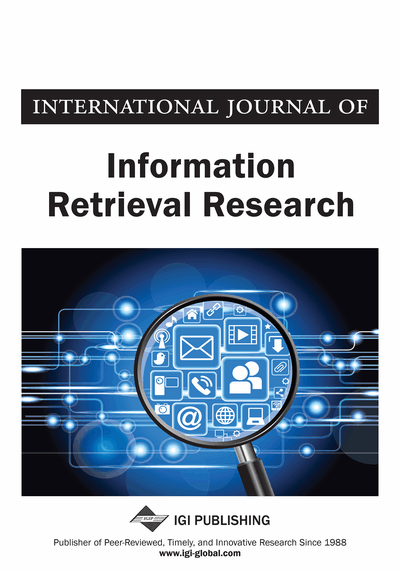# A Hybrid Classification Approach Based on Decision Tree and Naïve Bays Methods

Saed A. Muqasqas (Department of Computer Information Systems, Yarmouk University, Irbid, Jordan), Qasem A. Al Radaideh (Department of Computer Information Systems, Yarmouk University, Irbid, Jordan) and Bilal A. Abul-Huda (Department of Computer Information Systems, Yarmouk University, Irbid, Jordan)
DOI: 10.4018/IJIRR.2014100104
Available
\$37.50
No Current Special Offers

## Abstract

Data classification as one of the main tasks of data mining has an important role in many fields. Classification techniques differ mainly in the accuracy of their models, which depends on the method adopted during the learning phase. Several researchers attempted to enhance the classification accuracy by combining different classification methods in the same learning process; resulting in a hybrid-based classifier. In this paper, the authors propose and build a hybrid classifier technique based on Naïve Bayes and C4.5 classifiers. The main goal of the proposed model is to reduce the complexity of the NBTree technique, which is a well known hybrid classification technique, and to improve the overall classification accuracy. Thirty six samples of UCI datasets were used in evaluation. Results have shown that the proposed technique significantly outperforms the NBTree technique and some other classifiers proposed in the literature in term of classification accuracy. The proposed classification approach yields an overall average accuracy equal to 85.70% over the 36 datasets.
Article Preview
Top

## 1. Introduction

Data mining is the field that is concerned in extracting useful knowledge from large amount of data. Data mining employs several tasks and techniques toward extracting the knowledge including: classification, clustering, and association. Data classification is considered one of the most important techniques in data mining where in data classification a model is generated by a learning process of classification and then the model can be used for predication. Data Classification has contributed to many fields, such as medical diagnosis, remote sense, radar, etc (Sarkar and Sana, 2009, Haouari, et al., 2009; Friedman, et al., 1997).

There are several techniques that have been proposed and used for data classification such as the decision tree based techniques, Naïve Bays, Neural Networks, Genetic algorithms and many others (Han and Kamber, 2006).

A Naïve Bayes is a simple probabilistic classifier that is based on applying Bayes’ theorem for Thomas Bayes with strong independence assumptions. The Naïve Bayes classifier is widely used for its simplicity and traceability and it is considered a fast learner in comparison to other complex classification techniques (Langley, et al., 1992). Because of the simplicity of Naïve Bayes algorithm and the linear run time, it becomes a popular learning classifier for many data mining applications (Hall, 2007).

In Naïve Bays classifier, to predict the class label (Ci.) of a given instance (X), the classifier need to compute the posterior probability P(Ci|X) that an instance X = (x1, x2, x3, .., xn) belongs to the class Ci. The probability is computed using the following formula where xi is the value of attribute Ai and xn is the value of attribute An.

Where P(Ci) is the priori probability P(Ci) = |Ci,D|/|D|, where |Ci,D| is the number of instances of class Ci in the training dataset and |D| is the number of the instances in the training dataset.

Naïve Bayes algorithm can deal with continuous and nominal values. In addition Naïve Bayes has the most suitable dealing with complex and incomplete dataset (Soria, et al, 2011). This indicates that the Naïve Bayes has easy dealing with a number of features or classes and it is a fast learning algorithm that examines all its training dataset (Ratanamahatana & Gunopulos, 2003).

The decision tree based algorithms such as C4.5, ID3, or CART are known methods can handle the real world datasets efficiently (Han and Kamber, 2006). The C4.5 algorithm was proposed and designed in the nineties of the last century by Quinlan (1986) after 10 years of designing ID3. C4.5 builds the decision tree in a recursive fashion where it computes the Gain ratio measure for each attribute in the dataset then selects the best attribute that has the maximal Gain ratio to be the root node of the decision tree. The attribute of the maximum gain ratio is picked up for splitting the dataset to reduce the needed information to predict a given instance in the resulting attribute’s partition.

Kohavi (1996) proposed an approach called NBTree algorithm (Naïve Bayes Tree) which combines the Naïve Bayes and Decision Tree methods. Jiang and Li (2011) proposed another algorithm called C4.5-NB which is an enhancement of the NBTree algorithm.

NBTree and C4.5-NB, have proven their efficiency on different datasets, however, NBTree learning process is considered complex, in which a Naive Bayes classifier is built on each leaf node of the resulted decision tree. On the other side, C4.5-NB uses a simple approach in the learning process but with less accuracy compared with NBTree. Therefore, there is a need to build a hybrid classifier that is simple and has a better accuracy in comparison to C4.5-NB and NBTree algorithms.

## Complete Article List

Search this Journal:
Reset
Open Access Articles: Forthcoming
Volume 12: 4 Issues (2022): Forthcoming, Available for Pre-Order
Volume 11: 4 Issues (2021): 2 Released, 2 Forthcoming
Volume 10: 4 Issues (2020)
Volume 9: 4 Issues (2019)
Volume 8: 4 Issues (2018)
Volume 7: 4 Issues (2017)
Volume 6: 4 Issues (2016)
Volume 5: 4 Issues (2015)
Volume 4: 4 Issues (2014)
Volume 3: 4 Issues (2013)
Volume 2: 4 Issues (2012)
Volume 1: 4 Issues (2011)
View Complete Journal Contents Listing